# 转置卷积（Transpose Convolution）¶

## 一、转置卷积提出背景¶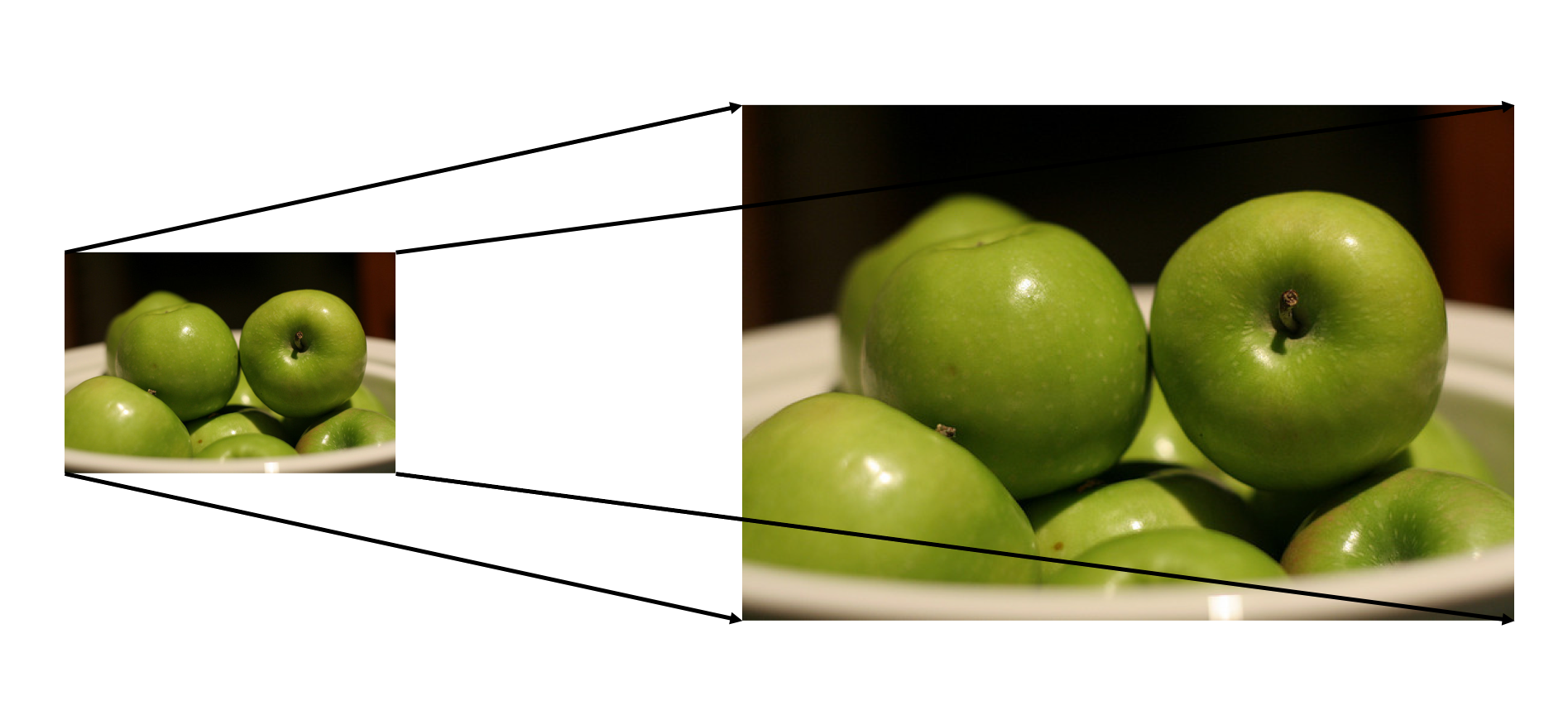## 二、转置卷积及其应用¶

• 在DCGAN，生成器将会用随机值转变为一个全尺寸(full-size)的图片，这个时候就需要用到转置卷积。

• 在语义分割中，会使用卷积层在编码器中进行特征提取，然后在解码层中进行恢复为原先的尺寸，这样才可以对原来图像的每个像素都进行分类。这个过程同样需要用到转置卷积。经典方法如：FCN和Unet

• CNN的可视化：通过转置卷积将CNN中得到的特征图还原到像素空间，以观察特定的特征图对哪些模式的图像敏感。

## 三、转置卷积与标准卷积的区别¶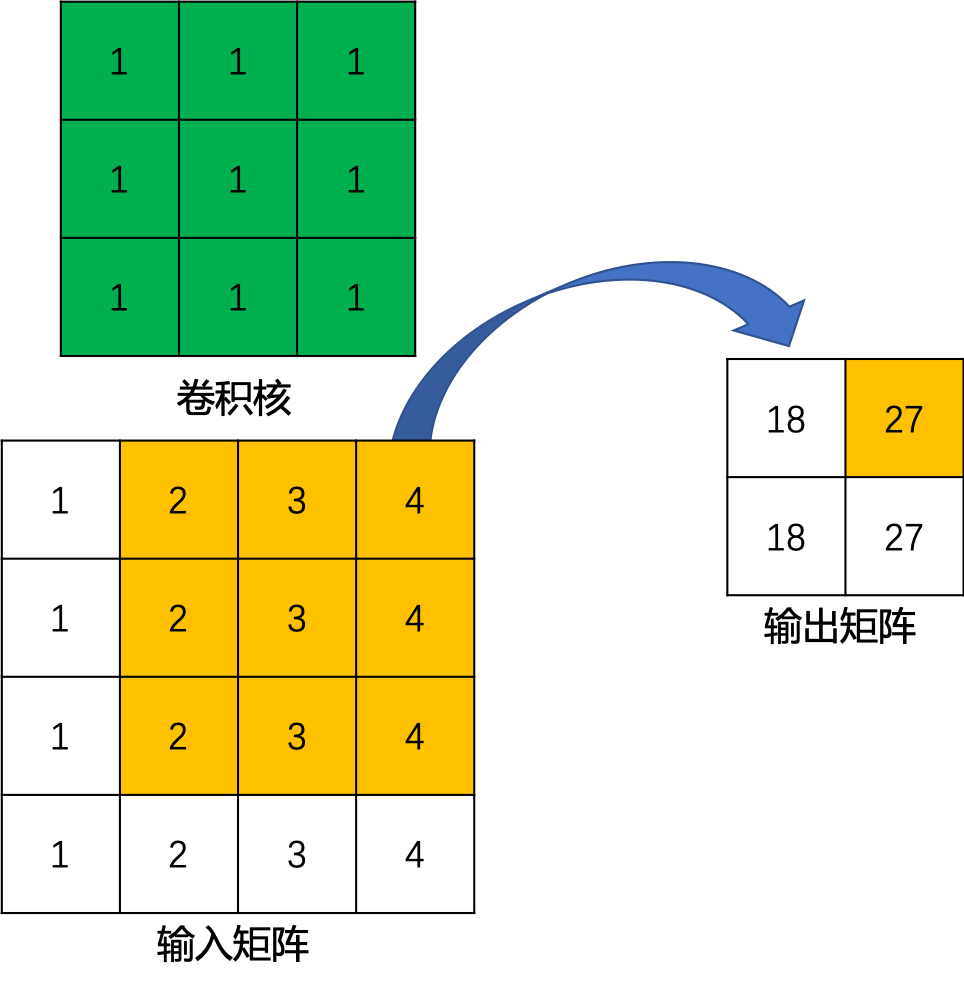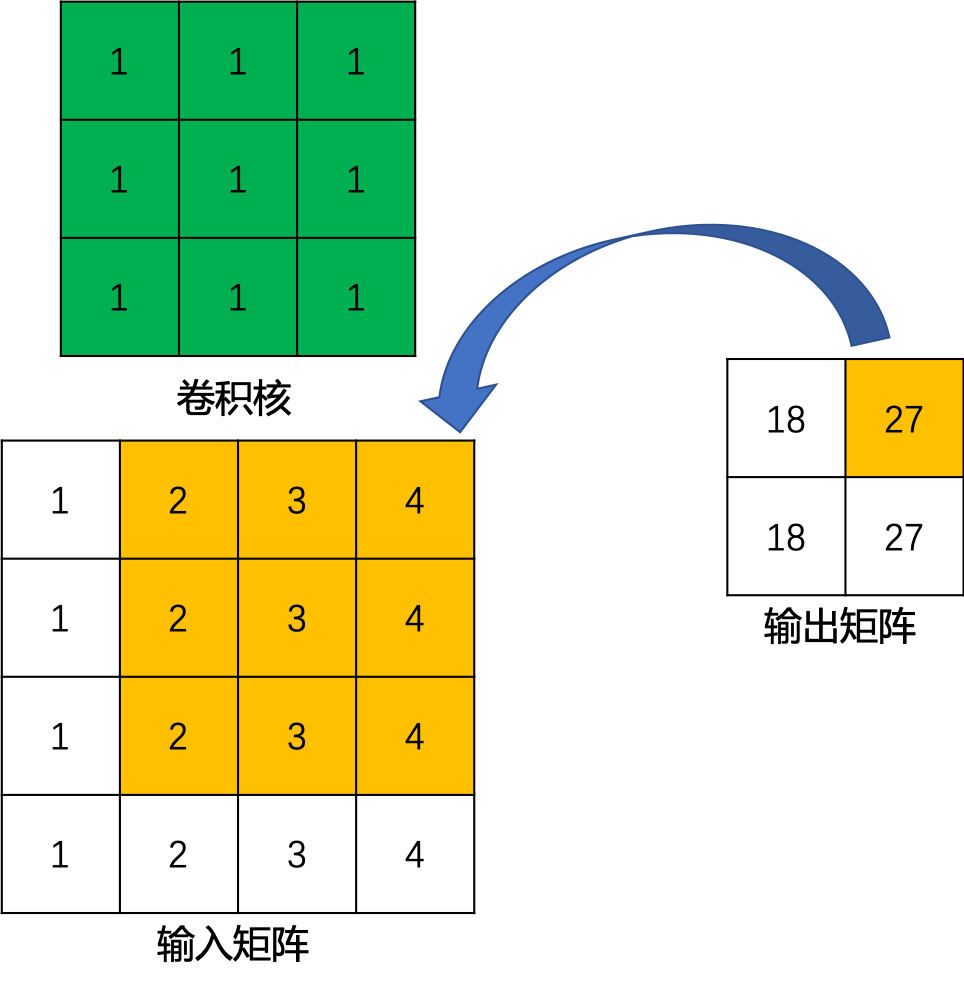## 四、转置卷积数学推导¶

$\begin{split} input=\left[\begin{array}{ccc} x_1 & x_2 & x_3 & x_4 \\ x_6 & x_7 & x_8 & x_9 \\ x_{10} & x_{11} & x_{12} & x_{13} \\ x_{14} & x_{15} & x_{16} & x_{17} \end{array}\right] \end{split}$

$\begin{split} kernel=\left[\begin{array}{ccc} w_{0,0} & w_{0,1} & w_{0,2} \\ w_{1,0} & w_{1,1} & w_{1,2} \\ w_{2,0} & w_{2,1} & w_{2,2} \end{array}\right] \end{split}$

$\begin{split} output=\left[\begin{array}{ccc} y_0 & y_1 \\ y_2 & y_3 \end{array}\right] \end{split}$

$\begin{split} X=\left[\begin{array}{ccc} x_1 \\ x_2 \\ x_3 \\ x_4 \\ x_6 \\ x_7 \\ x_8 \\ x_9 \\ x_{10} \\ x_{11} \\ x_{12} \\ x_{13} \\ x_{14} \\ x_{15} \\ x_{16} \\ x_{17} \end{array}\right] \end{split}$
$\begin{split} Y=\left[\begin{array}{ccc} y_0 \\ y_1 \\ y_2 \\ y_3 \end{array}\right] \end{split}$

$Y = CX$

$\begin{split} \scriptsize{ C=\left[\begin{array}{ccc} w_{0,0} & w_{0,1} & w_{0,2} & 0 & w_{1,0} & w_{1,1} & w_{1,2} & 0 & w_{2,0} & w_{2,1} & w_{2,2} & 0 & 0 & 0 & 0 & 0 \\ 0 & w_{0,0} & w_{0,1} & w_{0,2} & 0 & w_{1,0} & w_{1,1} & w_{1,2} & 0 & w_{2,0} & w_{2,1} & w_{2,2} & 0 & 0 & 0 & 0 \\ 0 & 0 & 0 & 0 & w_{0,0} & w_{0,1} & w_{0,2} & 0 & w_{1,0} & w_{1,1} & w_{1,2} & 0 & w_{2,0} & w_{2,1} & w_{2,2} & 0 \\ 0 & 0 & 0 & 0 & 0 & w_{0,0} & w_{0,1} & w_{0,2} & 0 & w_{1,0} & w_{1,1} & w_{1,2} & 0 & w_{2,0} & w_{2,1} & w_{2,2} \end{array}\right] } \end{split}$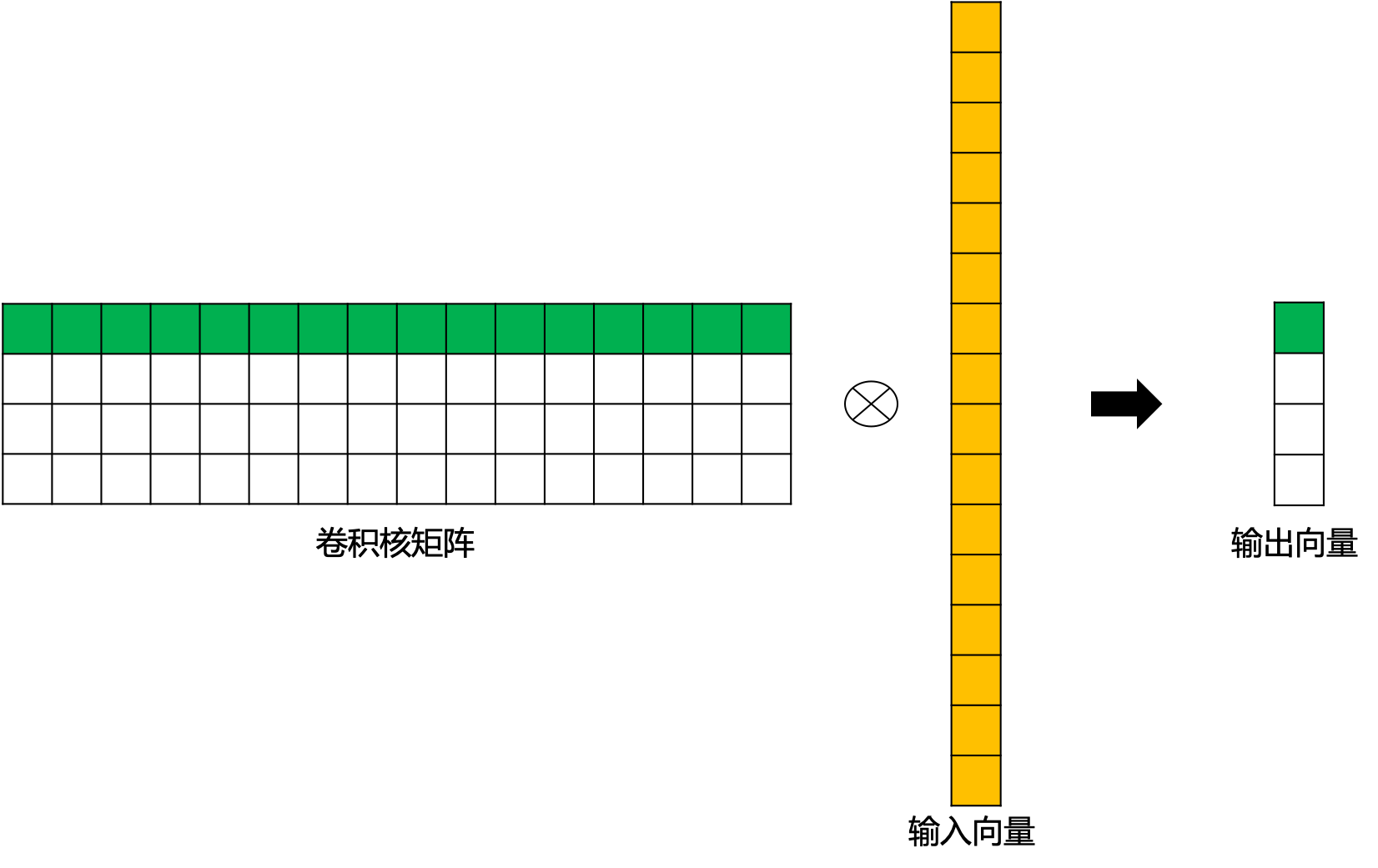$X = C^TY$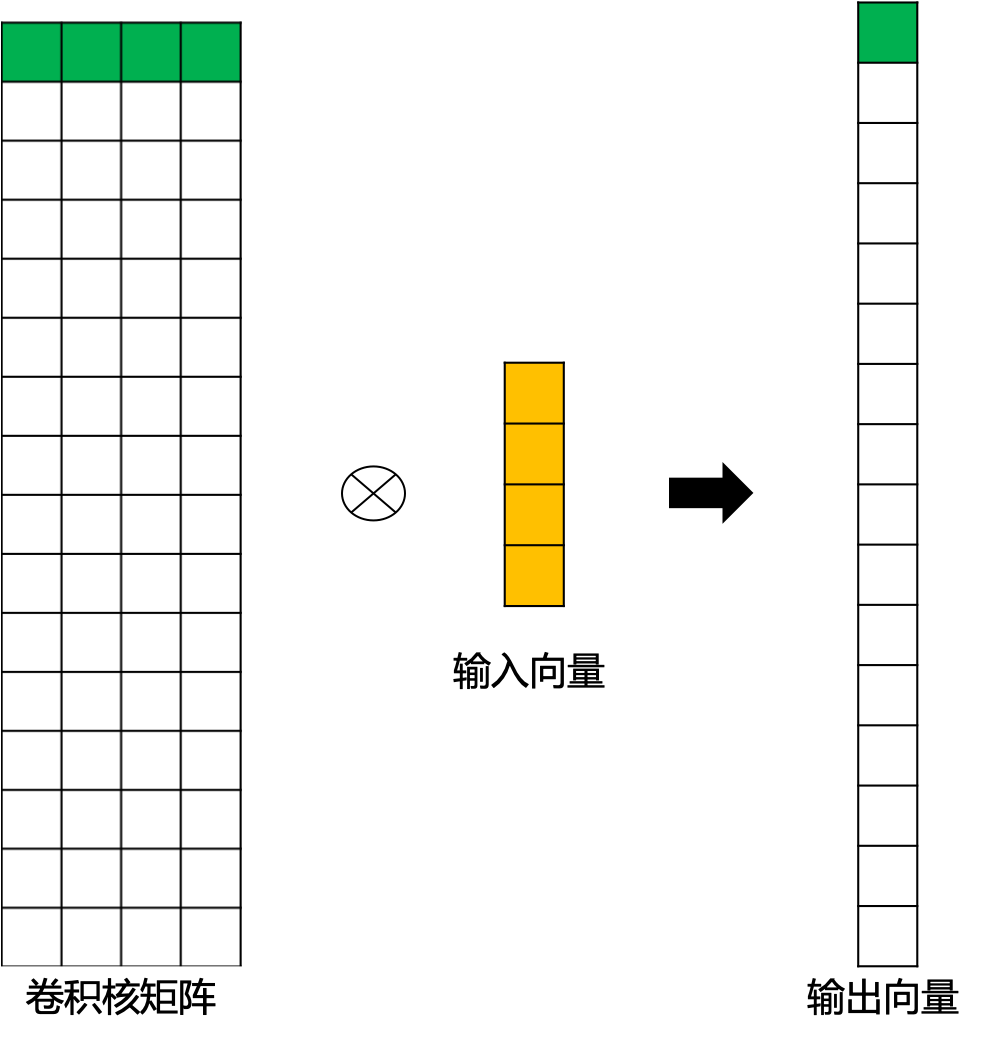## 五、转置卷积输出特征图尺寸¶

• stride=1的转置卷积

$\begin{split} \scriptsize{ C=\left[\begin{array}{ccc}w_{0,0} & w_{0,1} & w_{0,2} & 0 & w_{1,0} & w_{1,1} & w_{1,2} & 0 & w_{2,0} & w_{2,1} & w_{2,2} & 0 & 0 & 0 & 0 & 0 \\0 & w_{0,0} & w_{0,1} & w_{0,2} & 0 & w_{1,0} & w_{1,1} & w_{1,2} & 0 & w_{2,0} & w_{2,1} & w_{2,2} & 0 & 0 & 0 & 0 \\0 & 0 & 0 & 0 & w_{0,0} & w_{0,1} & w_{0,2} & 0 & w_{1,0} & w_{1,1} & w_{1,2} & 0 & w_{2,0} & w_{2,1} & w_{2,2} & 0 \\0 & 0 & 0 & 0 & 0 & w_{0,0} & w_{0,1} & w_{0,2} & 0 & w_{1,0} & w_{1,1} & w_{1,2} & 0 & w_{2,0} & w_{2,1} & w_{2,2} \end{array}\right] } \end{split}$

$\begin{split} output=\left[\begin{array}{ccc}y_0 & y_1 \\y_2 & y_3\end{array}\right] \end{split}$

$\begin{split} Y=\left[\begin{array}{ccc}y_0 \\ y_1 \\y_2 \\ y_3\end{array}\right] \end{split}$

$\begin{split} \scriptsize{ C^Ty'= \left[\begin{array}{ccc} w_{0,0}y_0 & w_{0,1}y_0+w_{0,0}y_1 & w_{0,2}y_0+w_{0,1}y_1 & w_{0,2}y_1 \\ w_{1,0}y_0+w_{0,0}y_2 & w_{1,1}y_0+w_{1,0}y_1+w_{0,1}y_2+w_{0,0}y_3 & w_{1,2}y_0+w_{1,1}y_1+w_{0,2}y_2+w_{0,1}y_3 & w_{1,2}y_1+w_{0,2}y_3 \\ w_{2,0}y_0+w_{1,0}y_2 & w_{2,1}y_0+w_{2,0}y_1+w_{1,1}y_2+w_{1,0}y_3 & w_{2,2}y_0+w_{2,1}y_1+w_{1,2}y_2+w_{1,1}y_3 & w_{2,2}y_1+w_{1,2}y_3 \\ w_{2,0}y_2 & w_{2,1}y_2+w_{2,0}y_3 & w_{2,2}y_2+w_{2,1}y_3 & w_{2,2}y_3 \end{array}\right] } \end{split}$

$\begin{split} input=\left[\begin{array}{ccc} 0 & 0 & 0 & 0 & 0 & 0 \\ 0 & 0 & 0 & 0 & 0 & 0 \\ 0 & 0 & y_0 & y_1 & 0 & 0 \\ 0 & 0 & y_2 & y_3 & 0 & 0 \\ 0 & 0 & 0 & 0 & 0 & 0 \\ 0 & 0 & 0 & 0 & 0 & 0 \end{array}\right] \end{split}$

$\begin{split} kernel‘=\left[\begin{array}{ccc} w_{2,2} & w_{2,1} & w_{2,0} \\ w_{1,2} & w_{1,1} & w_{1,0} \\ w_{0,2} & w_{0,1} & w_{0,0} \end{array}\right] \end{split}$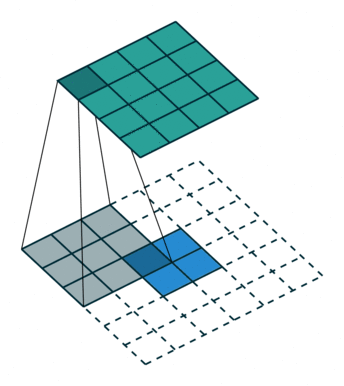$o' = i'+(k-1)$

• stride>1的转置卷积

$\begin{split} Y=\left[\begin{array}{ccc}y_0 \\ y_1 \\y_2 \\ y_3\end{array}\right] \end{split}$

$\begin{split} \scriptsize{ C^Ty'=\left[\begin{array}{ccc} w_{0,0}y_0 & w_{0,1}y_0 & w_{0,2}y_0+w_{0,0}y_1 & w_{0,1}y_1 & w_{0,2}y_1\\ w_{1,0}y_0 & w_{1,1}y_0 & w_{1,2}y_0+w_{1,0}y_1 & w_{1,1}y_1 & w_{1,2}y_1\\ w_{2,0}y_0+w_{0,0}y_2 & w_{2,1}y_0+w_{0,1}y_2 & w_{2,2}y_0+w_{2,0}y_1+w_{0,2}y_2+w_{0,0}y_3 & w_{2,1}y_1+w_{0,1}y_3 & w_{2,2}y_1+w_{0,2}y_3\\ w_{1,0}y_2 & w_{1,1}y_2 & w_{1,2}y_2+w_{1,0}y_3 & w_{1,1}y_3 & w_{1,2}y_3\\ w_{2,0}y_2 & w_{2,1}y_2 & w_{2,2}y_2+w_{2,0}y_3 & w_{2,1}y_3 & w_{2,2}y_3\\ \end{array}\right] } \end{split}$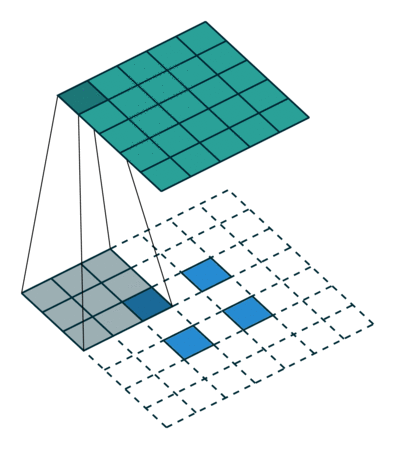$o' = s(i'-1)+k$